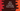# Java program to extract a substring from a string### Java program to extract a substring from a string :

In this Java programming tutorial, we will learn how to extract a substring from a user given string. The program will ask the user to enter a string and first and the second index of the substring. Then it will print out the substring of that string. Let’s take a look at the program :

### Java program :

``````import java.util.*;

public class Main {

public static void main(String[] args) {
//1
Scanner scanner = new Scanner(System.in);
String inputString;

//2
int startIndex;
int endIndex;

//3
System.out.println("Enter a string : ");
inputString = scanner.nextLine();

//4
System.out.println("Enter the first index of the substring : ");
startIndex = scanner.nextInt();

//5
System.out.println("Enter the second index of the substring : ");
endIndex = scanner.nextInt();

//6
char[] ch = new char[endIndex - startIndex + 1];
inputString.getChars(startIndex, endIndex + 1, ch, 0);

//7
System.out.println("Output : " + String.valueOf(ch));

}

}``````

### Explanation :

The commented numbers in the above program denote the step numbers below :

1. Create one Scanner object to read the user input values.Create one string inputString to store the user input string.
2. Create two variables to store the start and end index of the substring.
3. Ask the user to enter a string. Scan it and store it in inputString variable.
4. Ask the user to enter the first index , read and store it in startIndex variable.
5. Ask the user to enter the end index, read and store it in endIndex variable.
6. Create one character array ch to read and store the substring as character array.For this we are using getChars method.It takes 4 arguments : First one is the start index of the substring.
``````* Second one is the ending index of the string. If we pass _5_ as ending index, it will get the sub array up to _4th_ index.

* Third one is the character array to store the sub array.

* Fourth one is the starting index of the _character array_ where we are storing.``````
1. Finally, convert the character array to a string using String.valueOf() and print out the string.

### Sample output :

``````Enter a string :
hello
Enter the first index of the substring :
1
Enter the second index of the substring :
4
Output : ell

Hello World
Enter the first index of the substring :
1
Enter the second index of the substring :
6
Output : ello W``````